Neural Differential Equations
for Timeseries Forecasting
inby Stephan Sahm
founder ofJolin.io IT consulting

# Stephan Sahm• Founder ofJolin.io
• Full stack data science consultant
• Applied stochastics, uncertainty handling
• Big Data, High Performance Computing and Real-time processing

#Jolin.io

• We are based in Munich, working for Europe
• We focus on Julia consulting, high performance computing, machine learning and data processing.
• We build end-to-end solutions, including data architecture, MLOps, DevOps, GDPR, user interface, ...
• 10+ years experience in Data Science
5+ years in IT consulting
5+ years with Julia# Outline

Julia Introduction
Differential Equations within Neural Nets
Neural Nets within Differential Equations
Uncertainty Learning - Bayesian Estimation and DiffEq
Symbolic Regression
Benchmarks

#introduction

• Developed and incubated at MIT
• Version 1.0 in 2018
• Generic programming language
• Focus on applied mathematics
• Alternative to Python, R, Fortran, Matlab

### 3 Revolutions at once

30x-300x faster than Python
All in 1 language
Multimethods instead of inheritance simplify code-reuse and maintenance

### Where isused in production?

 Industries Pharma, Energy, Finance, Medicine & Bio-technology Fields of application Modeling/simulation, optimization/planning, Data Science, High Performance Computing, Big Data, Real-time

# Multimethods

intuitive generic programming - every function is an interface

        

struct Cat
name
description
end

struct Dog
name
end

kitty = Cat("Kitty", "the cute")
tiger = Cat("Tiger", "the strong")
bello = Dog("Bello")
charly = Dog("Charly")

mylittlestory(animal1, animal2) = [
describe(animal1)
describe(animal2)
meet(animal1, animal2)
]

describe(animal) = "This is $(animal.name)." meet(animal1, animal2) = "$(animal1.name) meets $(animal2.name)" describe(cat::Cat) = "Welcome$(cat.name), $(cat.description)." meet(cat::Cat, dog::Dog) = """$(cat.name) hisses at $(dog.name) and$(dog.name) takes off."""

mylittlestory(kitty, tiger)
mylittlestory(bello, charly)
mylittlestory(tiger, charly)




Method specialization works for arbitrary many arguments as well as if types and functions are in different packages

# Mandel-brot ExampleJulia 30x faster than Python & Numpy

100% Julia versus mixture Python & C

Python
Time:
1000x1000 in 4.5 min (267 sec)
200x200 in 10 sec
        

import numpy as np

def mandelbrot(c,maxiter):
z = c
for n in range(maxiter):
if abs(z) > 2:
return n
z = z*z + c
return 0

def mandelbrot_set(xmin = -0.74877, xmax = -0.74872,
ymin = 0.06505, ymax = 0.06510,
width = 1000, height = 1000,
maxiter = 2048):
r1 = np.linspace(xmin, xmax, width)
r2 = np.linspace(ymin, ymax, height)
n3 = np.empty((width,height))
for i in range(width):
for j in range(height):
n3[i,j] = mandelbrot(r1[i] + 1j*r2[j], maxiter)
return (r1, r2, n3)

import timeit
timeit.timeit(
lambda: mandelbrot_set(width=200, height=200),
number = 1
)




Optimising Python is generally depending on performant packages like Numpy & Numba.

better performance
=
better usage of performant packages

Julia
Time:
1000x1000 in 9 sek
200x200 in 0.4 sek
        

function mandelbrot(c, maxiter)
z = c
for n in 1:maxiter
if abs(z) > 2
return n
end
z = z*z + c
end
return 0
end

function mandelbrot_set(xmin = -0.74877, xmax = -0.74872,
ymin = 0.06505, ymax = 0.06510,
width = 1000, height = 1000,
maxiter = 2048)
r1 = range(xmin, xmax, length=width)
r2 = range(ymin, ymax, length=height)
n3 = zeros(Float32, width,height)
for i in 1:width
for j in 1:height
n3[i,j] = mandelbrot(r1[i] + r2[j]im, maxiter)
end
end
return (r1,r2,n3)
end

@time (plotx, ploty, plotz) = mandelbrot_set()
heatmap(plotx, ploty, plotz)




Optimising Julia can be done everywhere.

better performance
=
better usage of Julia itself

# Differential Equations within Neural Nets

Example Lotka-Volterra equations:
Dynamics of population of rabbits and wolves.
$$x^\prime = \alpha x - \beta x y$$ $$y^\prime = -\delta y + \gamma x y$$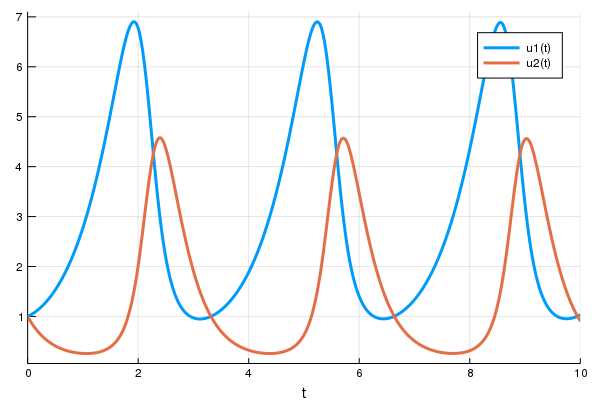Julia model

        

using DifferentialEquations
function lotka_volterra(du,u,p,t)
x, y = u
α, β, δ, γ = p
du = dx = α*x - β*x*y
du = dy = -δ*y + γ*x*y
end
u0 = [1.0, 1.0]
tspan = (0.0, 10.0)
p = [1.5, 1.0, 3.0, 1.0]
prob = ODEProblem(lotka_volterra,u0,tspan,p)



Solving and plotting
        

sol = solve(prob)
using Plots
plot(sol)




Source: https://julialang.org/blog/2019/01/fluxdiffeq/

Putting ODE into Neural Network Framework Flux.jl

loss function (let's say we want a constant number of rabbits)

        

using Flux, DiffEqFlux
p = [2.2, 1.0, 2.0, 0.4] # Initial Parameter Vector
params = Flux.params(p)
function predict_rd() # Our 1-layer "neural network"
# override with new parameters
solve(prob, Tsit5(), p=p, saveat=0.1)[1,:]
end
loss_rd() = sum(abs2, x-1 for x in predict_rd()




train for 100 epochs

        

data = Iterators.repeated((), 100)
Flux.train!(loss_rd, params, data, opt)




can be part of larger network

        

neuralnet = Chain(
Dense(28^2, 32, relu),
# requires an ODE of 32 params
p -> solve(prob, Tsit5(), p=p,
saveat=0.1)[1,:],
Dense(32, 10),
softmax)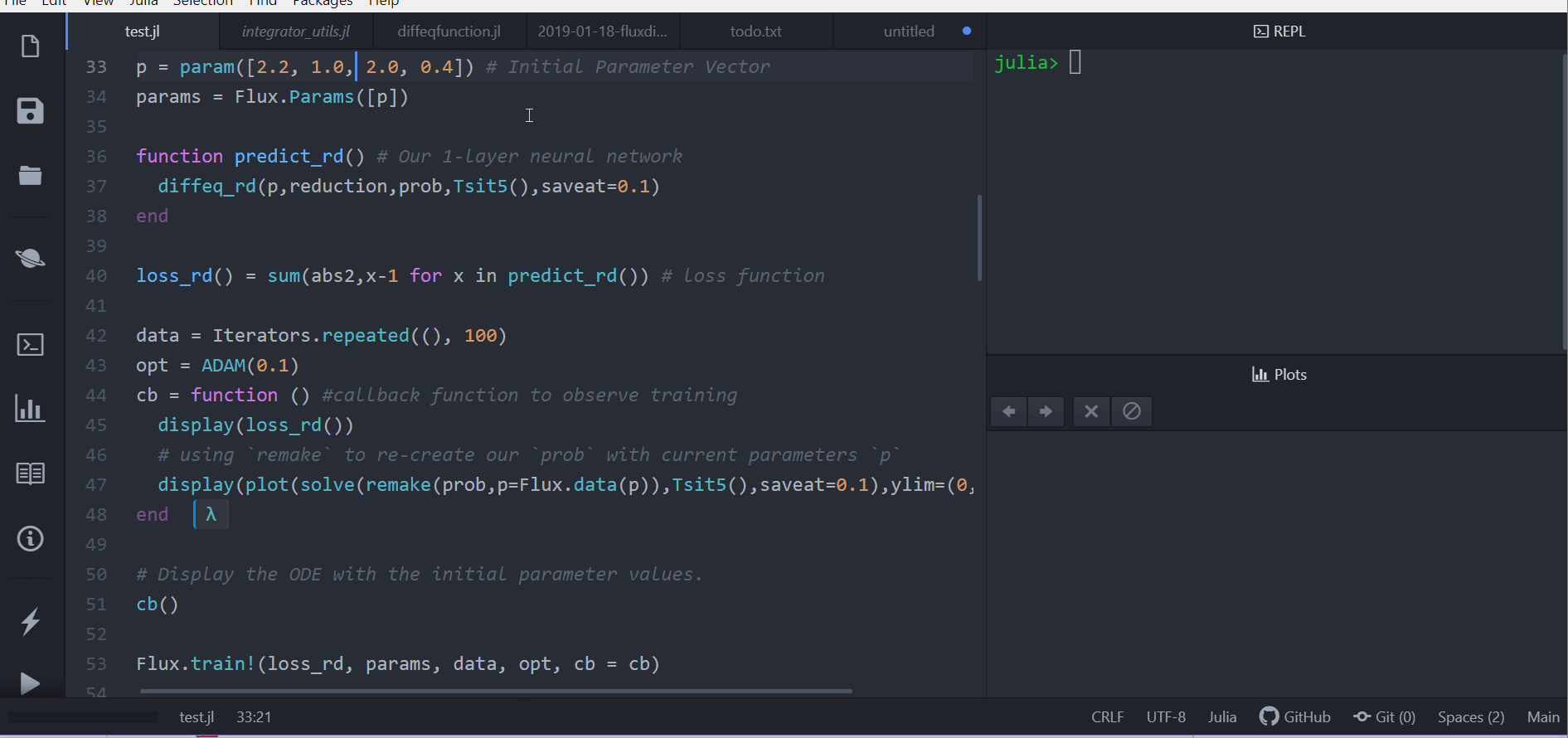# Neural Nets within Differential Equations

Ground Truth $u^\prime = A u^3$

        

using DifferentialEquations
function trueODEfunc(du,u,p,t)
true_A = [-0.1 -2.0
2.0 -0.1]
du .= true_A * u.^3
end

u0 = Float32[2.; 0.]
datasize = 30
tspan = (0.0f0, 1.5f0)

t = range(tspan,tspan,length=datasize)
prob = ODEProblem(trueODEfunc,u0,tspan)
ode_data = Array(solve(prob,Tsit5(),saveat=t))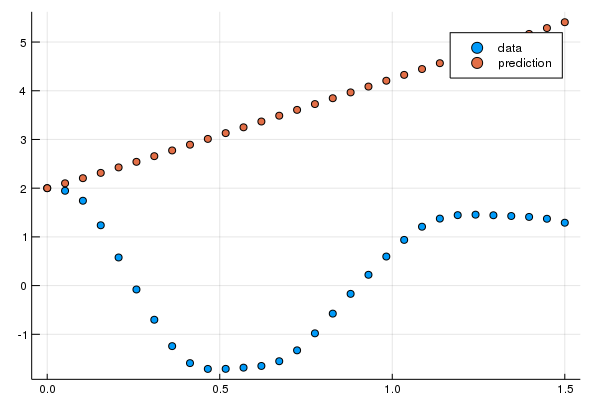Model $u^\prime$ with neural network.
(multilayer perceptron with 1 hidden layer and a tanh activation function)

        

using Flux, DiffEqFlux
dudt = Chain(x -> x.^3,
Dense(2, 50, tanh),
Dense(50, 2))

n_ode = NeuralODE(dudt, tspan, Tsit5(), saveat=t,
reltol=1e-7, abstol=1e-9)
ps = Flux.params(n_ode)

# Get the prediction using the correct initial condition
pred = n_ode(u0)




Source: https://julialang.org/blog/2019/01/fluxdiffeq/

plot

        

scatter(t, ode_data[1,:], label="data")
scatter!(t, pred[1,:], label="prediction")




train

        

data = Iterators.repeated((), 1000)
Flux.train!(loss_n_ode, ps, data, opt)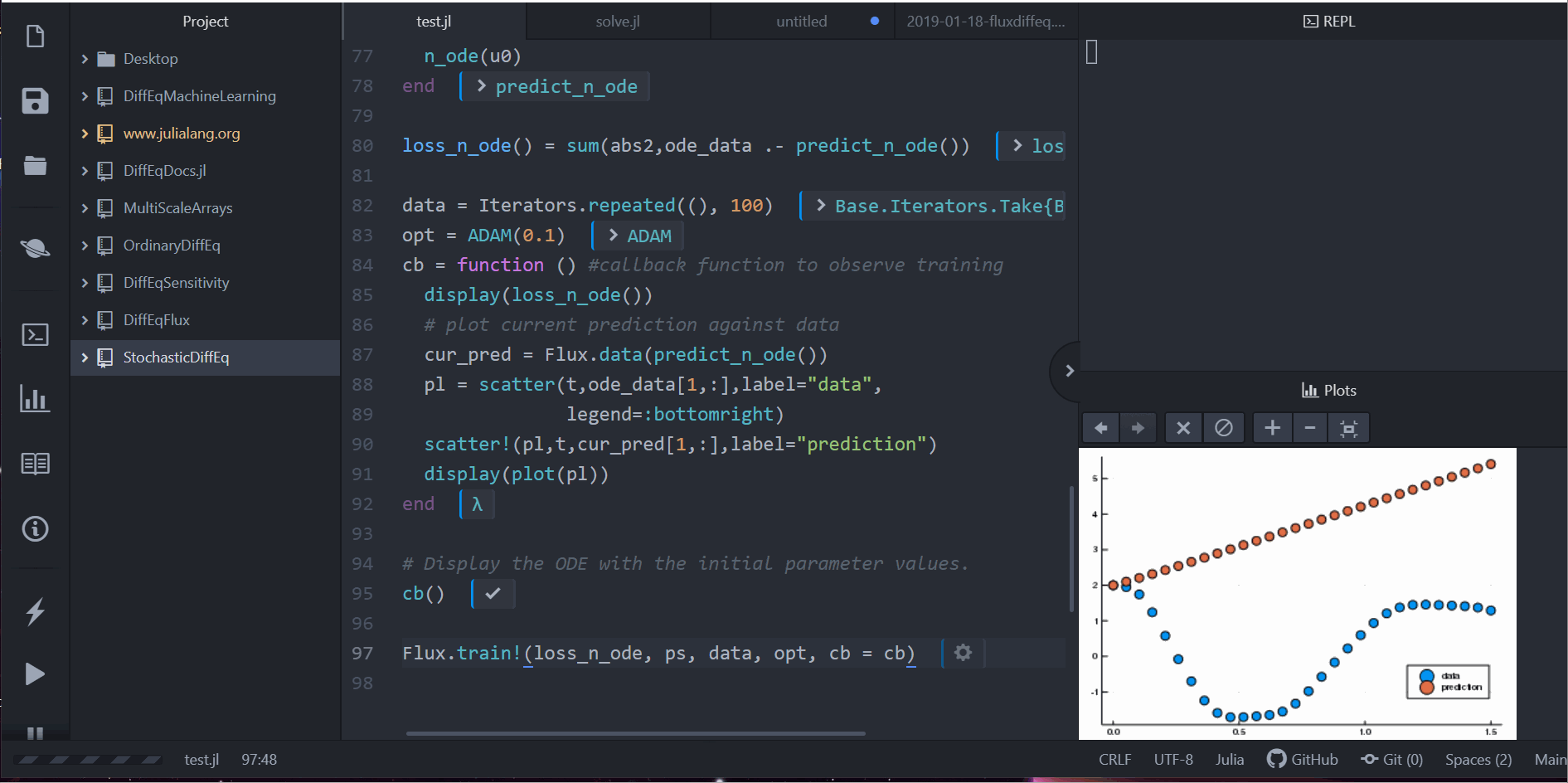# Neural Nets within Differential Equations

Alternative motivation for Neural Differential Equations: Generalization from Residual Nets.

Source: Neural Ordinary Differential Equations (Chen et al. 2019)

Residual Neural Network
discrete difference layers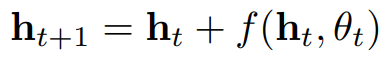Neural Ordinary Differential Equation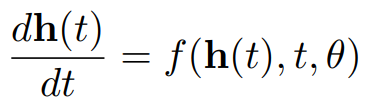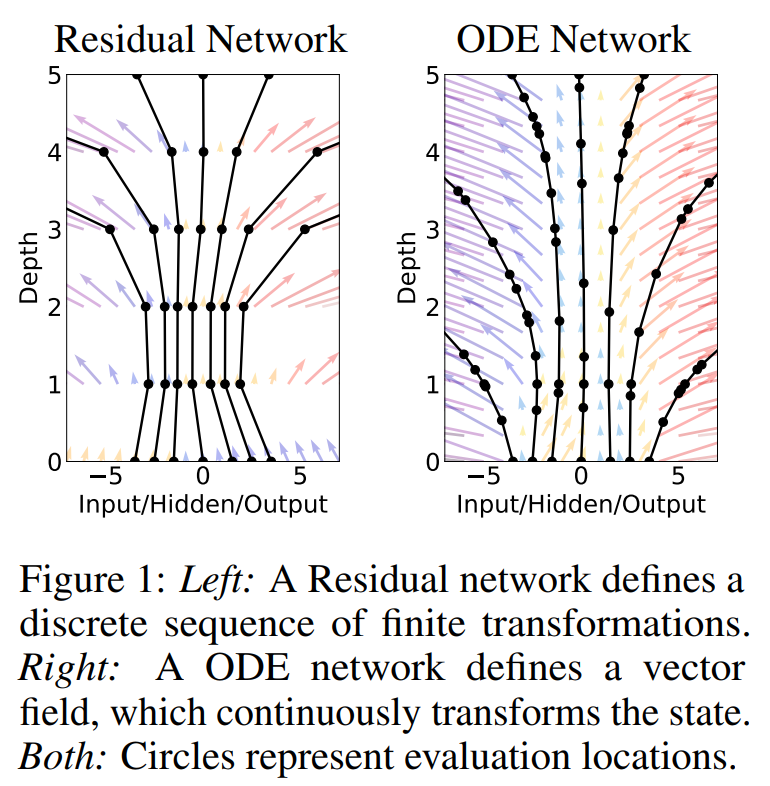# Uncertainty Learning - Bayesian Estimation and DiffEq

Let's assume noisy data

        

sol = solve(prob, Tsit5(), saveat=0.1)
odedata = (Array(sol) + 0.8
* randn(size(Array(sol))))
# Plot simulation & noisy observations
plot(sol, alpha=0.3)
scatter!(sol.t, odedata',
color=[1 2], label="")Let's assume we only have predator-data (wolves)

Probabilistic Model of the parameters

        

@model function fitlv(data::AbstractVector, prob)
# Prior distributions.
α ~ truncated(Normal(1.5, 0.5), 0.5, 2.5)
β ~ truncated(Normal(1.2, 0.5), 0, 2)
γ ~ truncated(Normal(3.0, 0.5), 1, 4)
δ ~ truncated(Normal(1.0, 0.5), 0, 2)
p = [α, β, γ, δ]

# Simulate Lotka-Volterra model but save only
# the second state of the system (predators).
predicted = solve(prob, Tsit5(), p=p, saveat=0.1, save_idxs=2)

# Observations of the predators.
σ ~ InverseGamma(2, 3)
data ~ MvNormal(predicted.u, σ^2 * I)
return nothing
end

# fit model only to predators (wolves)
model = fitlv(odedata[2, :], prob)




Source: https://turing.ml/dev/tutorials/10-bayesian-differential-equations/

Sample & plot

        

# Sample 3 independent chains.
chain = sample(model, NUTS(0.45), MCMCSerial(), 5000, 3, progress=false)
posterior_samples = sample(chain[[:α, :β, :γ, :δ]], 300, replace=false)

plot(legend=false)
for p in eachrow(Array(posterior_samples))
sol_p = solve(prob, Tsit5(), p=p, saveat=0.1)
plot!(sol_p, alpha=0.1, color="#BBBBBB")
end

# Plot simulation and noisy observations.
plot!(sol, color=[1 2], linewidth=1)
scatter!(sol.t, odedata', color=[1 2])# Symbolic Regression

Extract human readable formula from learned Neural Differential Equation

# Benchmarks

Source: Universal Differential Equations for Scientific Machine Learning (Rackauckas et al. 2021)

### Features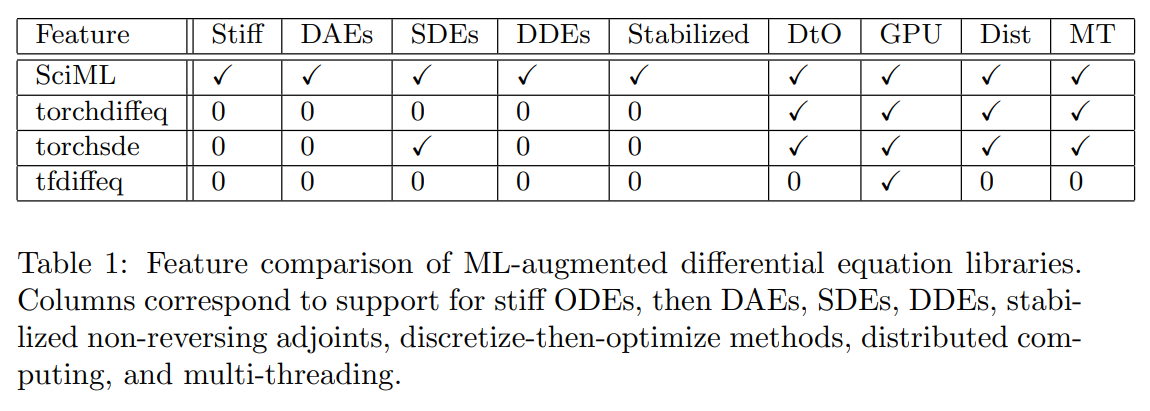### Speed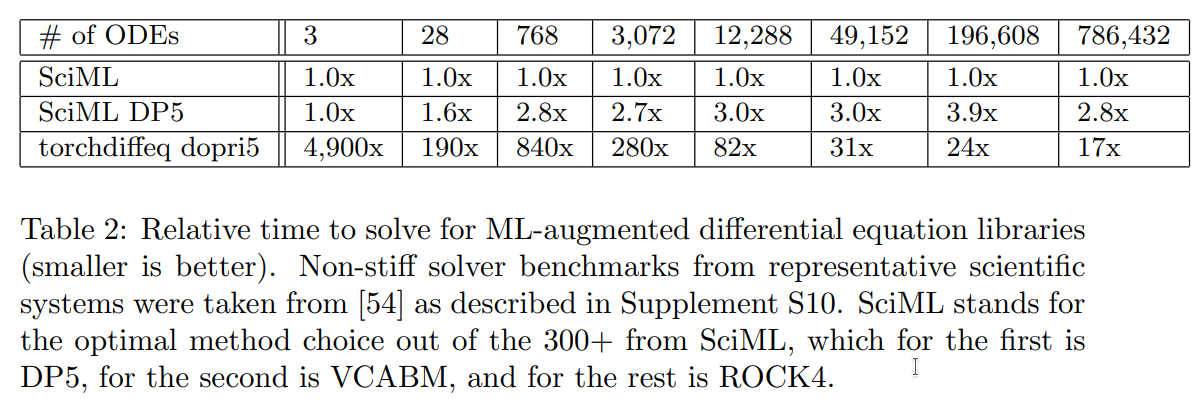“torchdiffeq’s adjoint calculation diverges on all but the first two examples due to the aforementioned stability problems.”

tfdiffeq (TensorFlow equivalent): “speed is almost the same as the PyTorch (torchdiffeq) code base (±2%)”

# Summary

Julia Introduction
• 30x-300x faster than Python
• 100% Julia
• Multimethods
Differential Equations within Neural Nets
• Think of an ODE as another layer for your Neural Network.
• Works with ODE, SDE, DDE, DAE, PDE.
Neural Nets within Differential Equations
• Model the derivative with a Neural Network.
• Generalization of Residual Networks.
• Works with ODE, SDE, DDE, DAE, PDE.
Uncertainty Learning - Bayesian Estimation and DiffEq
• Model randomness.
• Capture training uncertainty.
• Works with ODE, SDE, DDE, DAE, PDE.
Symbolic Regression